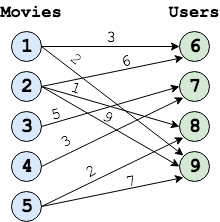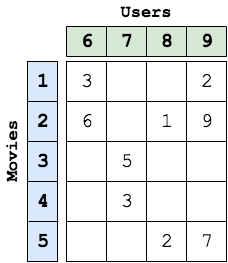# Matrix Completion

## Application Description

Matrix completion is an application which fills the missing entries in a partially observed or filled matrix. One example is the movie-ratings matrix in the Netflix Problem: Given a rating matrix in which entry (i,j) represents the rating given to movie i by user j if user has watched that movie, otherwise that entry will be missing in the matrix. Now the problem is to predict the values of the missing entires as accurately as possible in order to make good recommendations to the users to watch new movies.
The input to matrix completion is a bipartite graph. Figure 1 shows an example of a movie rating bipartite graph. Figure 2 shows the equivalent matix representation of the bipartite graph in Figure 1.The edges of the bipartite graph represent the filled entries in the matrix form of the graph and the edge values from node i to node j represents the rating given to movie i by user j. The numbers on the movie and user nodes represent the node IDs for the nodes of the graph as expected by this application is Galois. All the movie nodes (or nodes with out-going edges) must be laid out first with IDs 0 to N, followed by user nodes form N+1 to M, assuming that total number of movie nodes is N and total number of user nodes is M. In Figure 1, N is 5 and M is 4.We have a bulk-synchronous parallel (BSP) style distributed implementations of Stochastic Gradient Descent (SGD) (as shown in Figure 3) to solve matrix completion. The input graph is partitioned among hosts using one of the many partitioning policies provided by the CuSP. Each host computes SGD on the partitioned sub-graph assigned.

Synchronization of the gradient vectors occurs between BSP rounds. A node will average the vectors from all proxies that exist in the system for that node.

 1 2 3 4 5 6 7 8 9 10 11 12 13 14 foreach (MovieNode n: graph) { movie_LA = n.latent_array foreach (UserNode m: n's neighbor) { user_LA = m.latent_array new_rating = dotProduct(movie_LA, user_LA) error = edge_value - new_rating for(i: LATENT_ARRAY_SIZE) { prevMovie = movie_LA[i] prevUser = user_LA[i] movie_LA[i] -= stepsize * ( error * prevUser + lambda * prevMovie) user_LA[i] -= stepsize * ( error * prevMovie + lambda * prevUser) } } }

Figure 3: Pseudocode for SGD algorithm of matrix completion

## Performance

GraphTime (s)
8 Hosts32 Hosts
Amazon1570.2696.2

The table above shows the execution time SGD algorithm. The experiments were conducted on Stampede Cluster (Stampede2), which is connected through Intel Omni-Path Architecture (peak bandwidth of 100Gbps). Each node has 2 Intel Xeon Platinum 8160 Skylake CPUs with 24 cores per CPU and 192GB DDR4 RAM. We use up to 128 CPU machines, each with 48 threads.